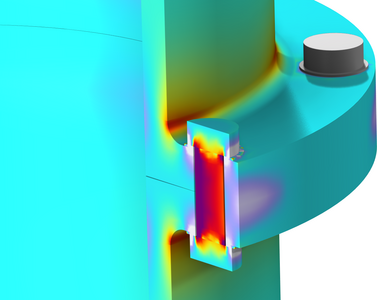# Bibliothèque d'Applications

## Prestressed Bolts in a Tube Connection

Application ID: 185

A tube connection consisting of a flange with eight prestressed bolts is subjected to a set of loads consisting of an internal pressure, an axial force, and an external bending moment.

The model domain consists of one quarter of the full geometry, since there are two planes of symmetry.

The study consists of two steps: prestressing the bolts and studying how the stress state in the tube and the bolts varies with the applied external load.

Both the given pretension force and the computed bolt forces of the cut bolts in the symmetry plane are automatically interpreted as if it were a full bolt, thus simplifying the modeling workflow.

A number of stress classification lines are then created, along which a stress linearization evaluation is performed. Stress linearization is a postprocessing technique where the stresses through a thin section in a solid model are represented by a constant membrane stress field and a linearly varying bending stress field. Membrane, bending, and peak stresses are reported and stress intensities are computed for each such stress classification line.